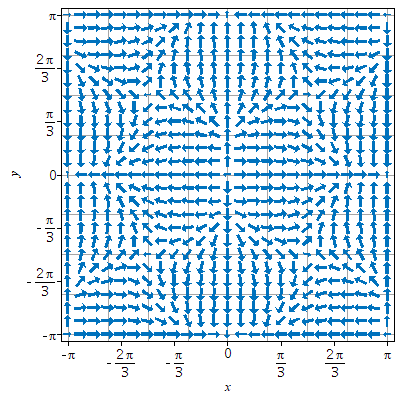# Question:How to change the placement of arrows in a 2-D/3-D quiver plot?

## Question:How to change the placement of arrows in a 2-D/3-D quiver plot?

Maple 2023

In an old question, @nm asked how to get my Maple output to look like I get with Mathematica. While the answer in that question was almost up to the mark, there remains a regret.

As the instance listed below shows, Maple, by default, draws arrows on a rectangular grid (rather than on a hexagonal mesh):

```  # Example of a three-dimensional vector field:
vf__2d := VectorCalculus:-VectorField([sin(x)*(cos(x) + cos(y)),
sin(y)*(cos(x) - cos(y))], 'cartesian'[x, y]):
# Example of a two-dimensional vector field:
vf__3d := VectorCalculus:-VectorField([1 - (sin(u - v) + sin(u - w)),
1 - (sin(v - w) + sin(v - u)),
1 - (sin(w - u) + sin(w - v))], 'cartesian'[u, v, w]):
# Phase portrait.
Student:-VectorCalculus:-PlotVector(vf__2d, (x, y) =~ -Pi .. Pi,
'grid' = [`\$`](25, 2),
'arrows' = 'THICK',
'fieldstrength' = log,
'color' = ColorTools:-Color("#0072BD"),
'axes' = "box"(*, …omitted…*));
= ```
``

Note that I have changed some of the options in order to make the layout of arrows more prominent.
However, according to the help page of Mma's `VectorPoints`, among the following methods of location generation, Mma by default uses Hexagonal for 2D field vectors and FaceCenteredCubic for 3D field vector:Here is a collection of different settings available in Mma:So if the requirement is to get the Maple's output looking like Mathematica's (see the beginning), the number and placement of vectors to plot should be thought of as well. In Maple, “the number of vectors” can be controlled by the plot (or plot3d) opinion grid, but how do I specify “the placement of vectors” (e.g., Mma's "Hexagonal" and "Mesh")?

Although there exists an arrow placement chapter in the documentation, randomly positioned arrows do not fit the bill. Is there any workaround?﻿